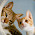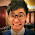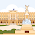2014/02/15

# 【中文 - 筆記】論四端 孟子 (語譯)【參考譯文 (2)】

「所以謂人皆有不忍人之心者，今人乍見孺子將人於井，皆有怵惕惻隱之心；非所以內交於孺子之父母也，非所以要譽於鄉黨朋友也，非惡其聲而然也。由是觀之，無惻隱之心，非人也；無羞惡之心，非人也；無辭讓之心，非人也；無是非之心，非人也。 惻隱之心，仁之端也；羞惡之心，義之端也；辭讓之心，禮之端也；是非之心，智之端也。」

「所以說每個人都有同情心，(譬如) 現在有人突然看見一個小孩子快要掉進井裡去了，(任何人) 都會有驚恐的樣子和憐憫的心；這不是為了要結交這小孩的父母，不是為了博取鄉親朋友間的名譽，也不是因為厭惡那小孩子的哭聲才這樣。由此看來，人如果沒有同情之心，簡直不是人；如果沒有羞恥之心，簡直不是人；如果沒有謙遜推讓的心，簡直不是人；如果沒有分辨正確與錯誤的心，簡直不是人。同情之心，是仁 (人與人相親) 的萌芽；羞恥之心，是義 (正義) 的萌芽；謙遜推讓的心，是禮的萌芽；分辨是非的心，是智的萌芽。」

「人之有是四端也，猶其有四體也。有是四端而自謂不能者，自賊者也；謂其君不能者，賊其君者也。凡有四端於我者，知皆擴而充之矣，若火之始然，泉之始達。苟能充之，足以保四海；苟不充之，不足以事父母。」

「人有這四種善性的萌芽，正好比他有手足四肢一樣是與生俱來的。有這四種萌芽卻認為自己不能行善的人，這是自暴自棄的人；認為他的君主不能行善的人，便是傷害他的君主。所有具有這四種萌芽的人，如果懂得把它們擴充起來，便會像火焰剛剛燒燃 (終必不可撲滅)；又像泉水剛剛流出 (終必匯為江河)。假使一個人能夠擴充這四種萌芽，便足以安定天下；假若不擴充這四種萌芽，就連父母也不行侍奉了。」

#### 28 意見:

1.2.good :)

3.good :)

1.謝！ :-)

2.作者已經移除這則留言。

4."非惡其聲而然也"的解释不应该是“不是厭惡他的名聲而這樣”，而应该跟上面的译文一样，是“也不是因為厭惡那小孩子的哭聲才這樣”。

1.感謝您的更正阿 :)
好少人會像你這樣認真研究網上語譯的準確性 (h)

2.我反而認為原來的才對

5.1.6.作者已經移除這則留言。

1.2.7.謝謝！ :-) :-) :-)

8.9.10.作者已經移除這則留言。

11.12.13.Very Good

14.15.非惡其聲而然也的意思是 也不是厭惡有不仁的名聲這才是對的

16.17.good thx :) :d :>) (o) 8-) \$-)

18.hi,:):):):):):):):):):):):):):):):):):):):):):):):):):):):):):):):):):):):):):):):):):):):):):):):):):):):):):):):):):):):):):):):):):):):):):):):):):):):):):):):):):):):):):):):):):):):):):):):):):):):):):):):):):):):):):):):):):):):):):):):):):):):):):):):):):):):):):):):):):):):):):):):):):):):):):):):):):):):):):):):):):):):):):):):):):)

19.20.21.【回覆須知】
※ 請注意網路禮儀，禁止口出惡言、灌水、廣告張貼
※ 為了加快網頁載入速度，請勿胡亂使用表情符號 (於留言框上方)
※ 與本文無關的留言請至「留言板
※ 勾選「通知我」可收到後續回覆的mail！

B. G. ﻿